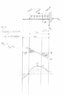# Shear Force and Moment Diagrams

TyErd

## Homework Statement

This cantilever confuses me so much. The negative point loads and negative distributed load. And is the other one that's -40kN/m moment/torque or something. My question is how do I do the shear force and bending moment diagrams of this. Am i suppose to redraw the diagram and make everything positive by changing the direction? can i even do that especially with the distributed load?

I don't really mind much for the numbers or anything its just the shape of the diagrams.
I've attached the diagram of the cantilever beam.

## Homework Equations

M=Fd where m=moment, f=force and d=perpendicular distance.

## The Attempt at a Solution

i have attempted and attached it and so can anyone tell me if this looks right?

#### Attachments

•scan0003.jpg
10.5 KB · Views: 413
Last edited:

Staff Emeritus
Homework Helper
You are confused because your problem diagram makes little sense. The applied torque should have units of kN-m, not kN/m; there is a difference. You also show an applied point load and an applied distributed load, both with negative magnitudes, yet each is drawn acting in opposite directions.

If you can provide a scan of the original problem, that would go a long way in resolving these contradictions.

TyErd
Yeah i realize the difference between kn-m and kn/m,, silly mistake by me but about the distributed load and point load, that's how the question is given. that is the original problem. negative values. i know its confusing but this is what was given to us. i can just simply switch the directions and make the values a positive right? even the distributed load? can i switch the direction of the torque and make it a positive?

is the shape of the bmd and sfd look right?

Staff Emeritus